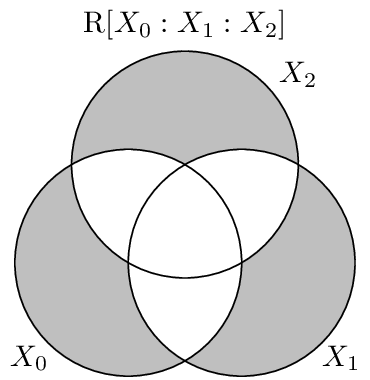# Residual Entropy¶

The residual entropy, or erasure entropy, is a dual to the Dual Total Correlation. It is dual in the sense that together they form the entropy of the distribution.

$\begin{split}\R{X_{0:n}} &= \sum \H{X_i | X_{\{0..n\}/i}} \\ &= -\sum_{x_{0:n} \in X_{0:n}} p(x_{0:n}) \log_2 \prod p(x_i|x_{\{0:n\}/i})\end{split}$

The residual entropy was originally proposed in [VW08] to quantify the information lost by sporatic erasures in a channel. The idea here is that only the information uncorrelated with other random variables is lost if that variable is erased.

If a joint distribution consists of independent random variables, the residual entropy is equal to the Entropy:

In : In : from dit.multivariate import entropy, residual_entropy


Another simple example is a distribution where one random variable is independent of the others:

In : In : d = dit.uniform(['000', '001', '110', '111'])

In : In : residual_entropy(d)
Out: 1.0

In : Out: 1.0


If we ask for the residual entropy of only the latter two random variables, the middle one is now independent of the others and so the residual entropy grows:

In : In : residual_entropy(d, [, ])
Out: 2.0

In : Out: 2.0


## Visualization¶

The residual entropy consists of all the unshared information in the distribution. That is, it is the information in each variable not overlapping with any other.## API¶

residual_entropy(dist, rvs=None, crvs=None, rv_mode=None)[source]

Compute the residual entropy.

Parameters
• dist (Distribution) – The distribution from which the residual entropy is calculated.

• rvs (list, None) – The indexes of the random variable used to calculate the residual entropy. If None, then the total correlation is calculated over all random variables.

• crvs (list, None) – The indexes of the random variables to condition on. If None, then no variables are condition on.

• rv_mode (str, None) – Specifies how to interpret rvs and crvs. Valid options are: {‘indices’, ‘names’}. If equal to ‘indices’, then the elements of crvs and rvs are interpreted as random variable indices. If equal to ‘names’, the the elements are interpreted as random variable names. If None, then the value of dist._rv_mode is consulted, which defaults to ‘indices’.

Returns

R – The residual entropy.

Return type

float

Raises

ditException – Raised if dist is not a joint distribution or if rvs or crvs contain non-existant random variables.